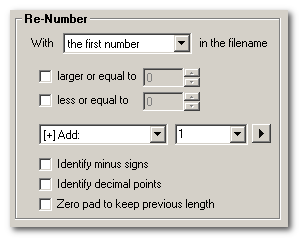This is a page from the Flash Renamer user manual.

# Re-NumberThe renumber function can pinpoint numbers inside your filenames and process them in ways that are not possible with other functions. It is very intuitive to use:

• First you select which numbers to process.

• Then you can, optionally, limit the numbers within a certain range, for example 0 to 100.

• Finally you select what to do with the number. You can replace it with something else, insert string before and after and even perform mathematical operations on the numbers!
Options
• Identify minus signs - If this is enabled, the program will look in front of each number. If it finds a hyphen (-) this is enterpeted as a minus sign belonging to the number. Otherwise this hyphen is simply ignored.
• Identify decimal points - Enabling this options enabled decimal fraction calculations in all arithmetic options in the Re-Number function. If a pediod or comma is found between two numbers it is enterpeted as a decimal point. Decimal fractions are automatically rounded to three decimals. You can change this in the advanced settings. Also note that since different countries use different decimal points, there are options available in the advanced settings that can customize how to work with decimal points.
• Zero pad to keep previous length - If you have a number like 001 and modify this number, it will become simply 1 unless you enable this option. (Not available when using dezimal fractions.)

Tip: To remove a number, simple choose "replace with" and leave the input field empty!